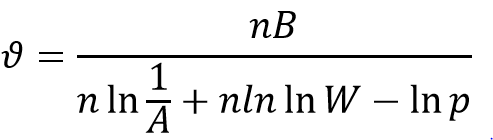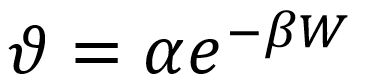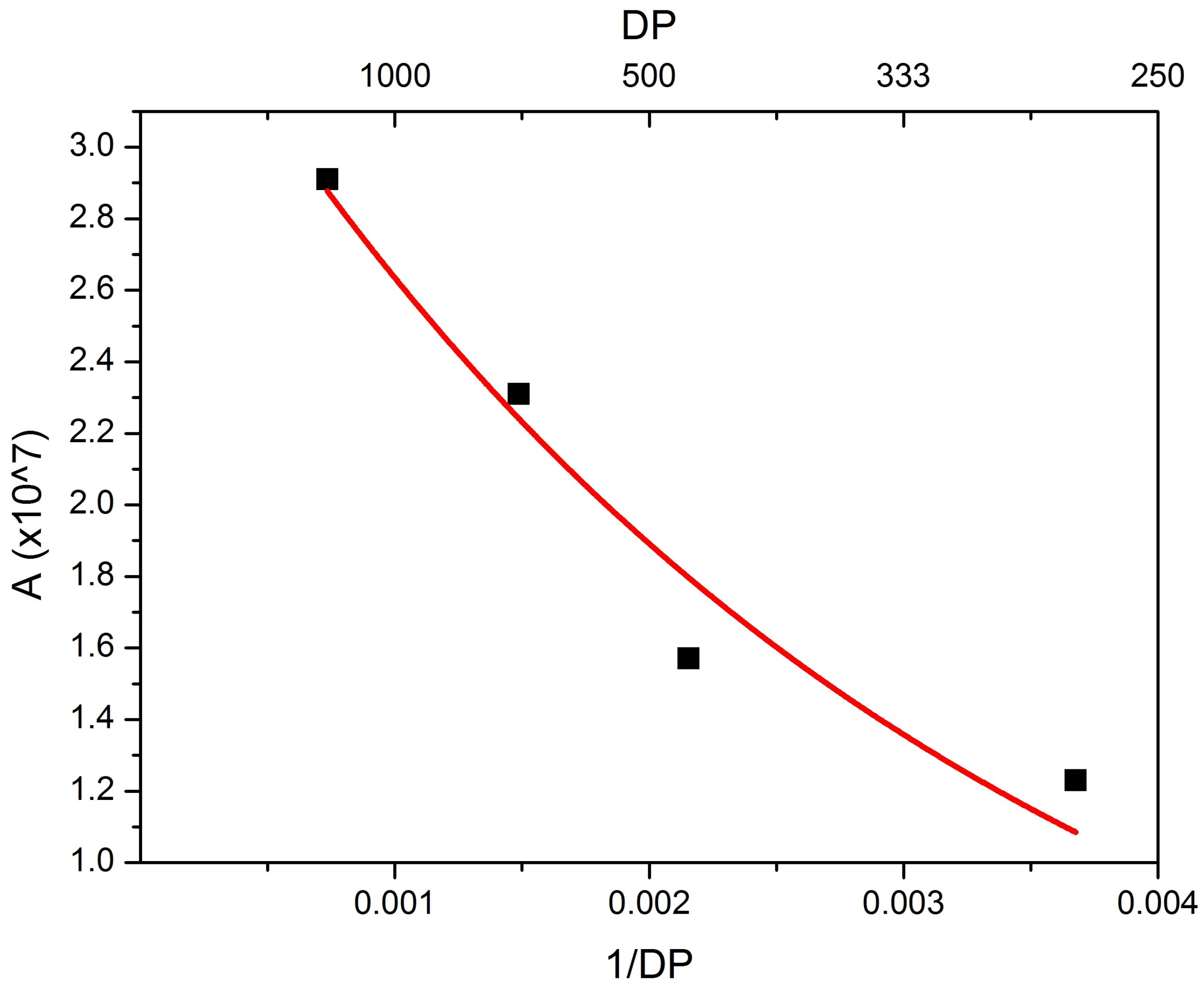# Influence of Paper Ageing on Transformer Failure

Ageing of paper insulation is a key parameter which determines the end of life for transformers. Much research has been conducted in trying to establish where the end of life point is, and it is common to relate this to the degree of polymerisation (DP) of the paper insulation. The DP is linked to the tensile strength (TS), and it is the TS of the paper which commonly leads to failures as the transformer cannot maintain its mechanical structure once the solid insulation is aged beyond a certain point.

This method of transformer failure is a long-term phenomenon. Transformers commonly exceed 20-30 year design life expectancy and there are transformers in the UK grid which are >80 years old. Other types of transformer failure occur more quickly, such as a dielectric failure resulting from bubble formation which can cause an instantaneous failure. Formation of moisture bubbles from the solid insulation causes a weakness in the dielectric strength of the insulation, and therefore it is important to avoid the generation of these bubbles.

Previous work by Pryzbylek had shown that bubble formation changes with reducing DP, with the temperature required to form bubbles being lower for more aged paper (paper of lower DP). A study was conducted to analyse how this effect can be described through a mathematical relationship.

The analysis is done for two different formulae used to calculate bubbling temperature. The first formula (1) is from the IEC / IEEE standards, and was first developed by Oommen in 2001. This formula is based on the isotherm behaviour of water and cellulose. Isotherms describe equilibrium conditions and so are not most suitable for explaining the dynamic bubbling process. An alternative formula (2) based on the enthalpy of desorption is also analysed. Both formulae were considered and were improved by including a dependence on DP. Figures 1 and 2 show how the parameters in (1) and (2) related to insulation ageing change with DP.(1)

where ϑ is the temperature of bubble inception (K), n is the adsorption topology constant, B is the specific energy of adsorption between moisture and paper (K), A is the frequency of effective adsorption events (torr-1), W is the moisture content of the paper insulation (g H2O / g paper), and p is the system pressure (torr).(2)

where α is the pre-exponential constant for the net sorption isosteric heat divided by the universal gas constant on a mass basis (K), and β is the temperature decay constant.Figure – Change of A from (1) with DP.Figure – Change of β from (2) with DP.

The study shows that the age of the paper insulation is crucial when considering the safe working limits of a transformer – a temperature limitation for a transformer at the start of life will not be suitable for through-life protection of the asset.

Within the IEC standard, 140°C is used as a limitation for bubbling. Table 1 shows the DP-moisture content values where this temperature is valid (using (2) with DP relationship from Figure 2), although typically a utility would apply an additional margin between the operational temperature and the limit which would increase the DP value shown.

Table – DP-Moisture conditions where 140°C is a valid limitation (DP rounded up to nearest 50 where bubble inception temperature is at least 1 K above 140°C).

 Moisture Content in Paper Degree of Polymerisation 0.5 100 1 200 2 700 4 No safe condition

The full paper is available at: Improvements to the Construction of Bubble Inception Formulae for Use with Transformer Insulation

Author: James Hill – The University of Manchester